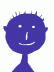## Some Perl Code In Memory of a Great Scientist

On August 21, 2021, famous Polish mathematician Andrzej Schinzel passed away at the age of 84. He was one of the great minds behind modern number theory. May he rest in peace. I have extended one of my CPAN modules relating to his work and dedicated the release to his memory.

I'll outline one of the insights credited to Beeger (1884-1965) and Schinzel. Suppose we want to factor an integer number of the form kn ± 1. We can use classical Algebra to factor rational polynomials xn ± 1. For example, x21 + 1 = (x + 1)(x2 - x + 1)(x6 - x5 + x4 - x3 + x2 - x + 1)(x12 + x11 - x9 - x8 + x6 - x4 - x3 + x +1). These factors always have integer coefficients, are called cyclotomic polynomials, and are "easy" to obtain. Evaluating these polynomials at x = k yields us a first partial factorization of our number.

To continue our example, we see that 221 + 1 = 3·3·43·5419, 321 + 1 = 4·7·547·682969, etc. Can we do better? Enter Aurifeuillean factorization. Aurifeuille, Le Lasseur and Lucas observed that some cyclotomic polynomials can be written in the form C2 - n·x·D2 = (C - √(n·x)D)(C + √(n·x)D) where n is a positive integer and C and D are again polynomials with integer coefficients. In algebraic terms, while irreducible over the field of Rationals ℚ, cyclotomic polynomials may be reducible over some extension field ℚ[√n]. Now if the square root of n·x happens to be an integer, that is when x is n times a square number, the formula above gets all integer, splitting a cyclotomic factor into two smaller integer factors. In short, Aurifeuille & Co. improve our factorization for some, but not all combinations of n and k.

For square-free n > 1, meaning n without square divisors, Lucas C,D polynomials can split one cyclotomic factor of nn - 1 if n ≡ 1 (mod 4), or of nn + 1 otherwise, into two smaller factors. Note that the base and the exponent are equal.

It turns out that these are special cases of something more profound. Beeger and Schinzel found the best currently known generalization. Essentially, they give us C,D polynomials covering more cases. To illustrate their range, let's compare some factorizations of 21st powers plus one. We leave out perfect powers, as kn21 can be better treated as an n21st power of k than as a 21st power of kn.

```+-----+------------+-----------------------------------------+
|  n  |  kind      |  factors of n^21+1                      |
+-----+------------+-----------------------------------------+
|     +------------+-----------------------------------------+
|  2  | cyclotomic |  3  3  43  5419                         |
|     +------------+-----------------------------------------+
|     | complete   |  3  3  43  5419                         |
+-----+------------+-----------------------------------------+
|     +------------+-----------------------------------------+
|  3  | cyclotomic |  4        7  547  682969                |
|     +------------+-----------------------------------------+
|     | Lucas      |  4     1  7  547  682969                |
|     +------------+-----------------------------------------+
|     | Schinzel   |  4     1  7  547  301    2269           |
|     +------------+-----------------------------------------+
|     | complete   |  2  2     7  547  7  43  2269           |
+-----+------------+-----------------------------------------+
|     +------------+-----------------------------------------+
|  5  | cyclotomic |  6     21    13021    290639881         |
|     +------------+-----------------------------------------+
|     | complete   |  2  3  3  7  29  449  7  43  127  7603  |
+-----+------------+-----------------------------------------+
|     +------------+-----------------------------------------+
|  6  | cyclotomic |  6     21    13021    290639881         |
|     +------------+-----------------------------------------+
|     | complete   |  2  3  3  7  29  449  7  43  127  7603  |
+-----+------------+-----------------------------------------+
|     +------------+-----------------------------------------+
|  7  | cyclotomic |  8        43  102943    15772610449     |
|     +------------+-----------------------------------------+
|     | Lucas      |  8        43  113  911  15772610449     |
|     +------------+-----------------------------------------+
|     | Schinzel   |  8        43  113  911  51031  309079   |
|     +------------+-----------------------------------------+
|     | complete   |  2  2  2  43  113  911  51031  309079   |
+-----+------------+-----------------------------------------+
```

As we can see, "Schinzel" polynomials, as I like to call them, help us with many large cyclotomic factors Lucas polynomials leave aside.

As I wanted to include Aurifeuillean factorization in my CPAN module Math::Polynomial::Cyclotomic for quite some time, I finally took the opportunity to do that. I implemented Lucas and "Schinzel" polynomials there as well as methods applying these to find algebraic factors of integer numbers of the form kn ± 1. Factoring large numbers can be hard—crypto-algorithms like RSA rely on that—, and a partial factorization can be the crucial step to reduce the problem to a feasible magnitude. Curiously, Lucas C,D polynomials seem to be rare in computer algebra libraries and Schinzel's results even rarer. At least CPAN now has some of these. I intend to add a Raku version later. A treasure trove of other number-theoretic functions can be found in Dana Jacobsen's Math::Prime::Util, including some that are used in M::P::Cyclotomic.

Andrzej Schinzel published more than 200 research papers and was the expert for number-theoretic aspects of polynomials. I dedicate the latest release of Math::Polynomial::Cyclotomic to his memory.

## ReferencesBlogging about Mathematics, Programming Languages, Open-Source, Privacy, Security, Board Games, Bicycles, Classical Music, and more.